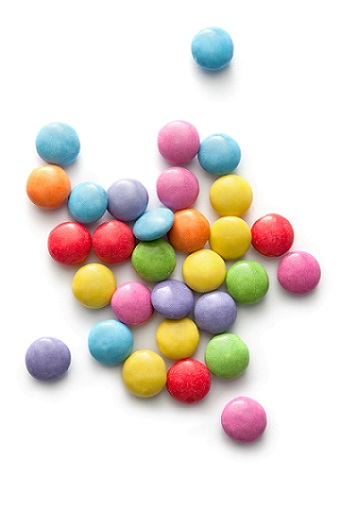Science, Maths & Technology

### Become an OU studentEveryday maths 2

Start this free course now. Just create an account and sign in. Enrol and complete the course for a free statement of participation or digital badge if available.

# 4.1 Writing a quantity of an amount as a fraction

Writing a quantity of an amount as a fraction might sound complicated, but it’s actually very logical. Look at the example below.Figure _unit2.4.2 Figure 6 Smarties in different colours

In Figure 6, what fraction of Smarties are red?

## Extract _unit2.4.1

=

To express the fraction of Smarties that are red, you simply need to count the red Smarties (4) and the total number of Smarties (30) Since there are 4 red Smarties out of 30 altogether, the fraction is . It is worth noting here that this could also be written as 4/30.

You may well be asked to give an answer as a fraction in it’s simplest form, which leads you nicely to the next section.